# Problem Statement 1 The pump in the system shown below is used to transport water from...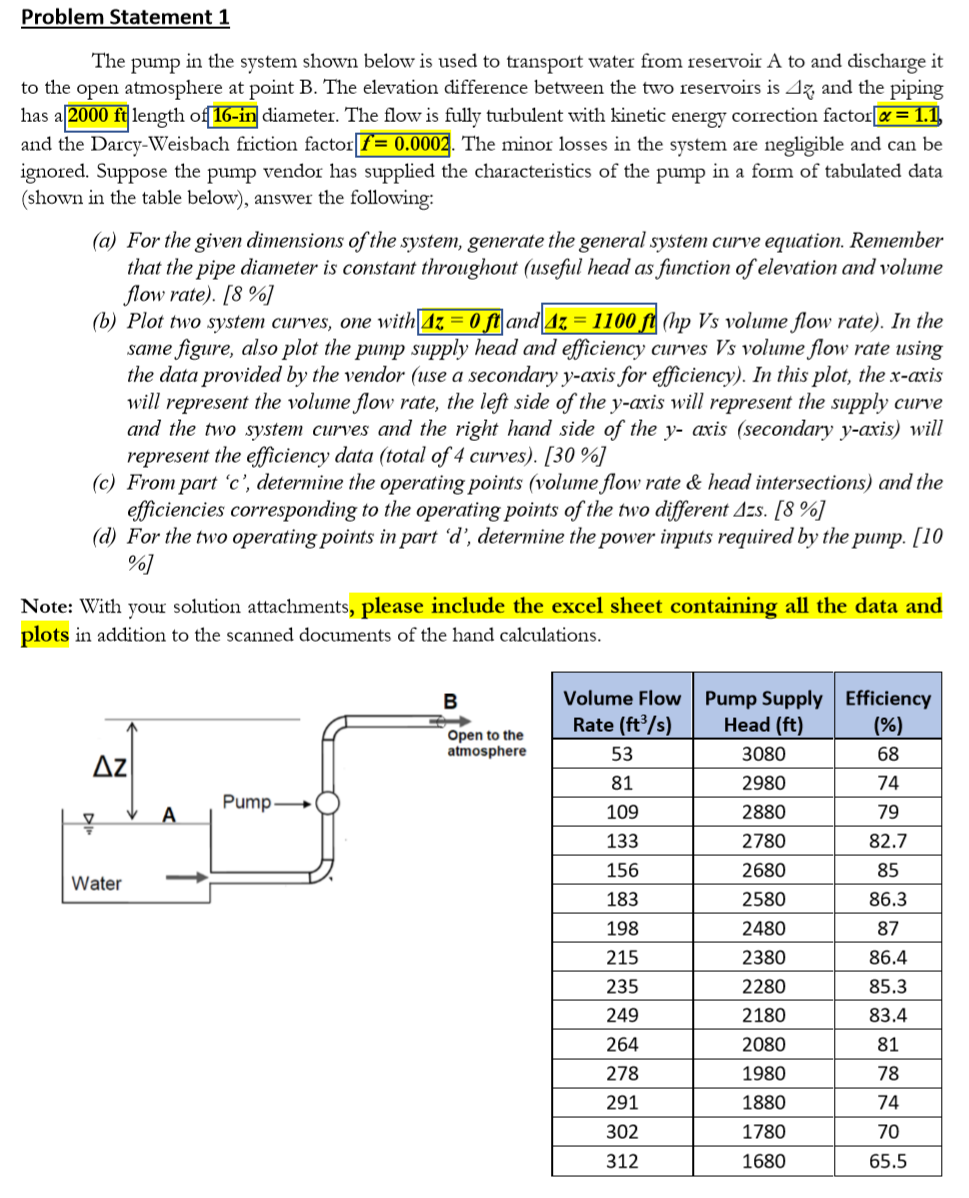Problem Statement 1 The pump in the system shown below is used to transport water from reservoir A to and discharge it to the open atmosphere at point B. The elevation difference between the two reservoirs is Az, and the piping has a 2000 ft length of 16-in diameter. The flow is fully turbulent with kinetic energy correction factor = 1.1 and the Darcy-Weisbach friction factor f = 0.0002. The minor losses in the system are negligible and can be ignored. Suppose the pump vendor has supplied the characteristics of the pump in a form of tabulated data (shown in the table below), answer the following: (a) For the given dimensions of the system, generate the general system curve equation. Remember that the pipe diameter is constant throughout (useful head as function of elevation and volume flow rate). [8 %] (b) Plot two system curves, one with|4z = 0 ft and Az = 1100 ft (hp Vs volume flow rate). In the same figure, also plot the pump supply head and efficiency curves Vs volume flow rate using the data provided by the vendor (use a secondary y-axis for efficiency). In this plot, the x-axis will represent the volume flow rate, the left side of the y-axis will represent the supply curve and the two system curves and the right hand side of the y- axis (secondary y-axis) will represent the efficiency data (total of 4 curves). [30 %] (c) From part 'c', determine the operating points (volume flow rate & head intersections) and the efficiencies corresponding to the operating points of the two different Azs. (8 %] (d) For the two operating points in part d', determine the power inputs required by the %] Note: With your solution attachments, please include the excel sheet containing all the data and plots in addition to the scanned documents of the hand calculations. pump. [10 B Volume Flow Rate (ft3/s) 53 Open to the atmosphere Az Pump A 81 109 133 Pump Supply Efficiency Head (ft) (%) 3080 68 2980 74 2880 79 2780 82.7 2680 85 2580 86.3 2480 87 2380 86.4 2280 85.3 2180 83.4 Water 156 183 198 215 235 249 264 278 2080 1980 1880 1780 1680 81 78 74 70 291 302 312 65.5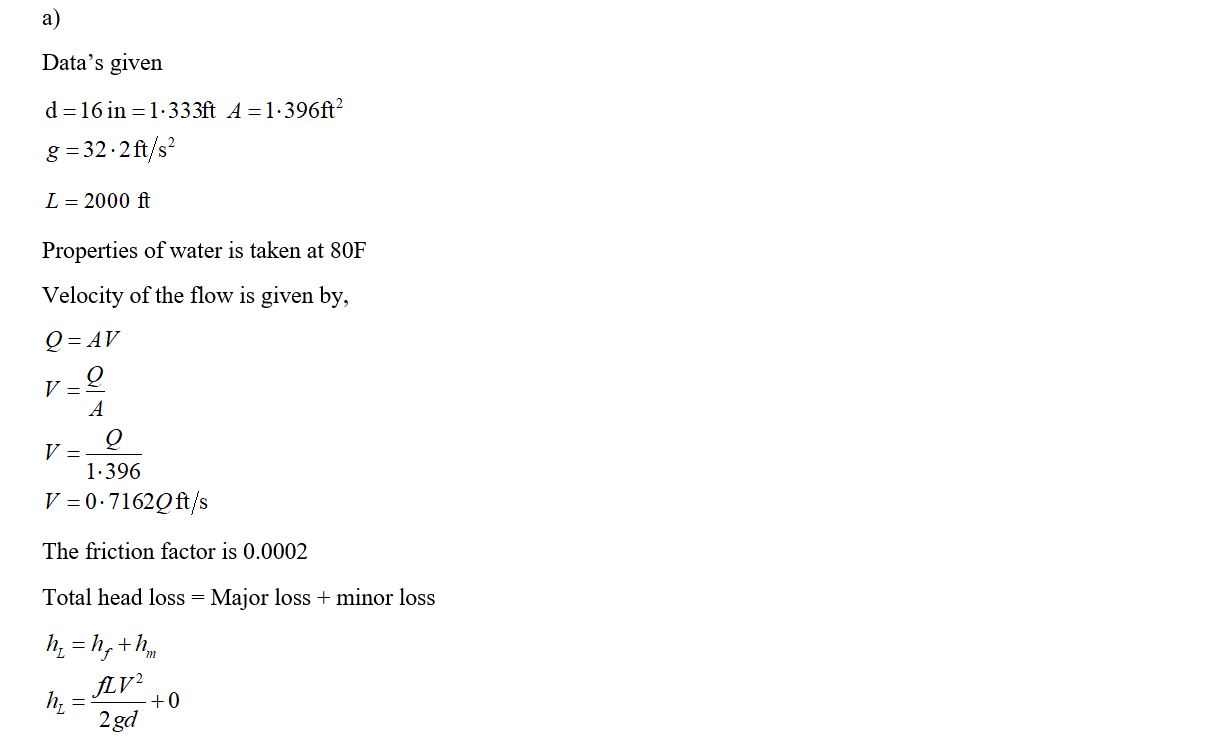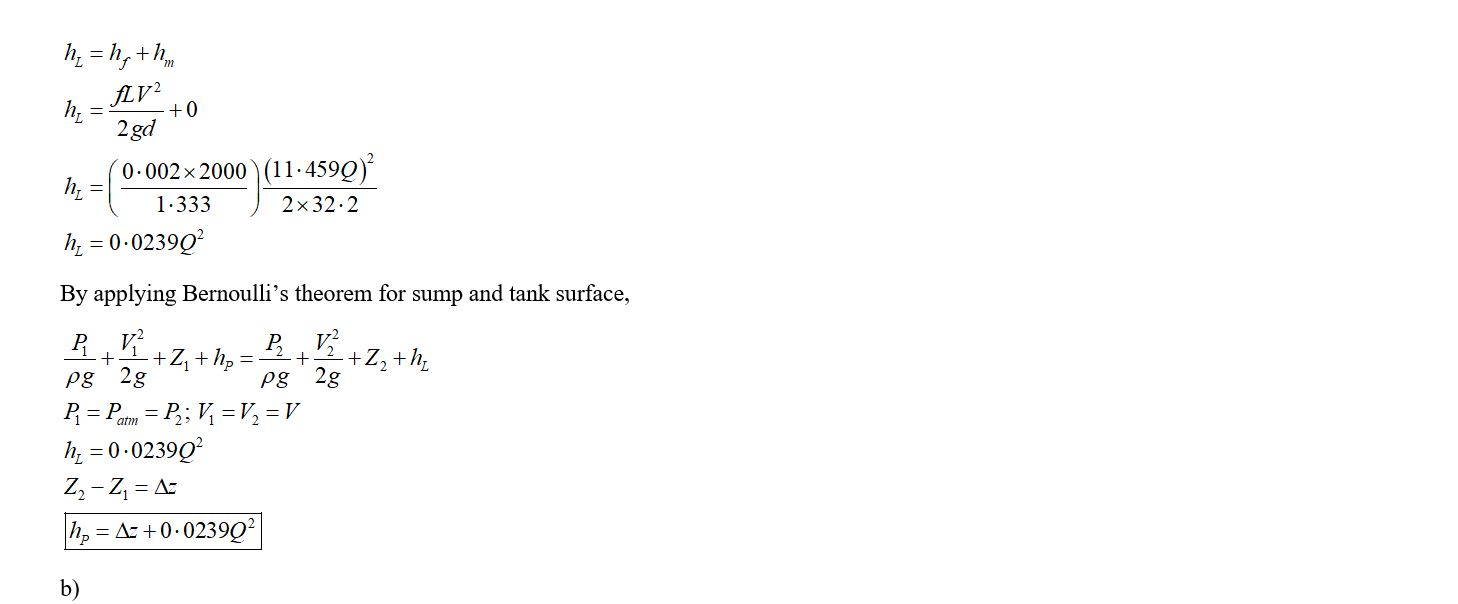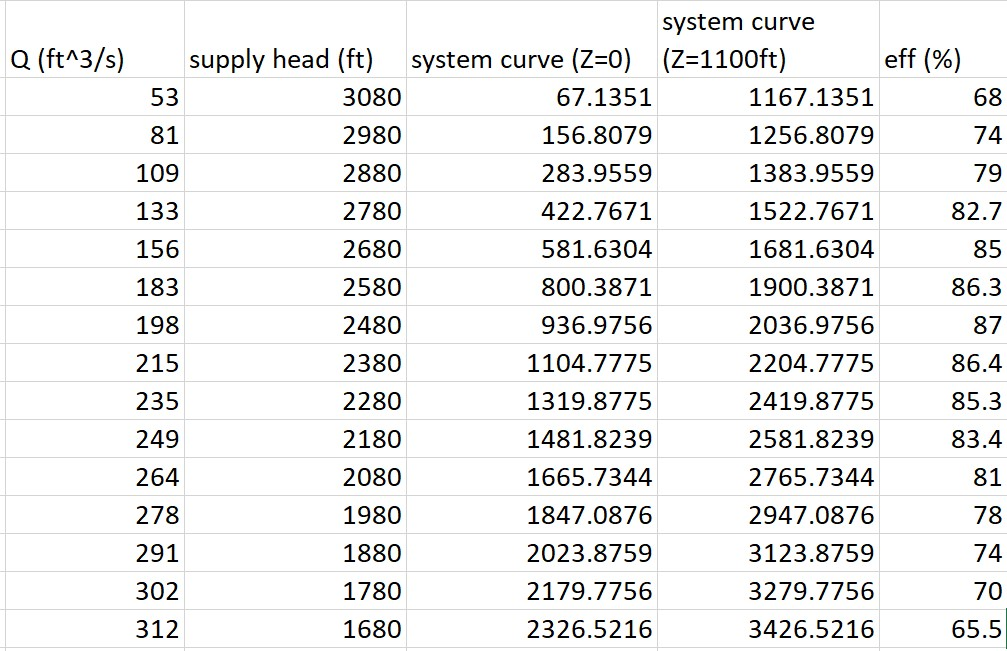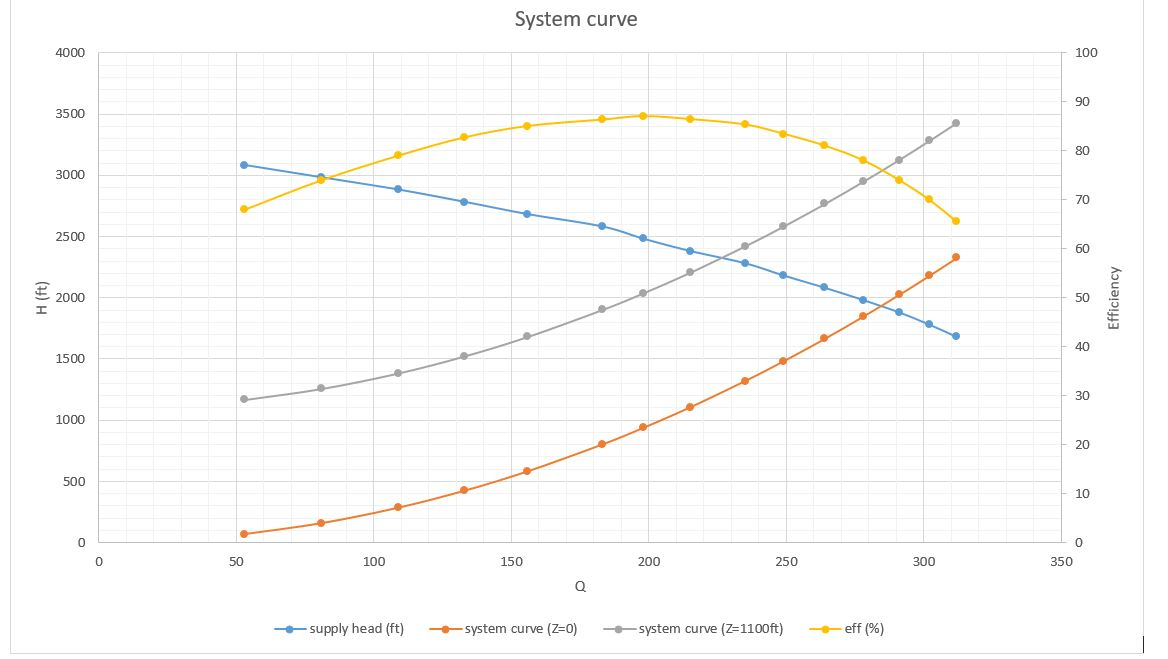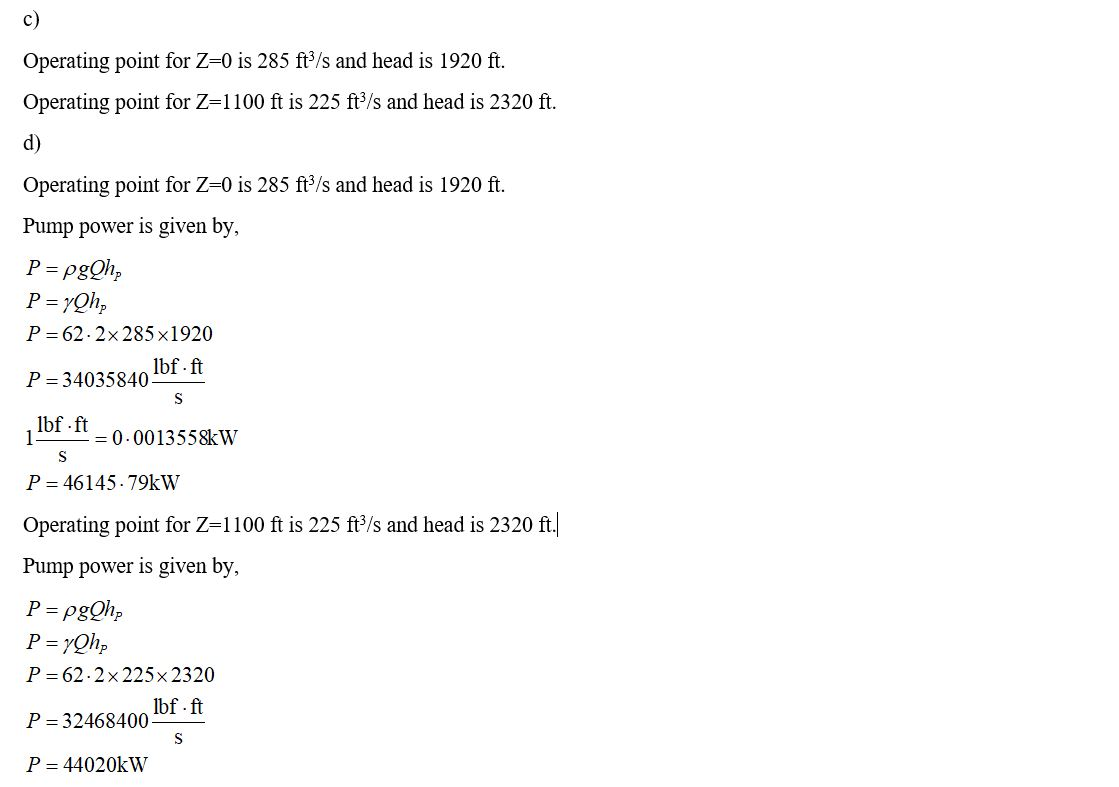#### Earn Coin

Coins can be redeemed for fabulous gifts.

Similar Homework Help Questions
• ### 4.45 A two-tank pump/pipe system has a head requirement of 23.4 ft at a flow rate of 100 gpm. The...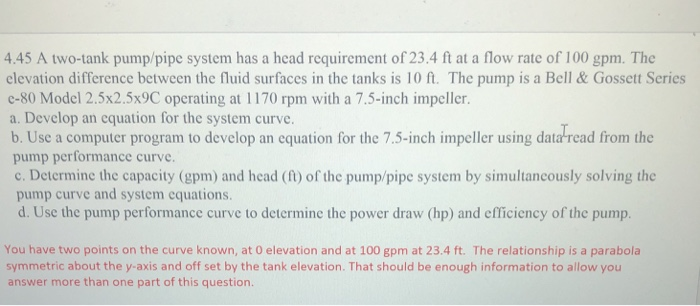4.45 A two-tank pump/pipe system has a head requirement of 23.4 ft at a flow rate of 100 gpm. The elevation difference between the fluid surfaces in the tanks is 10 ft. The pump is a Bell & Gossett Series e-80 Model 2.5x2.5x9C operating at 1170 rpm with a 7.5-inch impeller. a. Develop an cquation for the system curve. b. Use a computer program to develop an equation for the 7.5-inch impeller using data read from the pump performance curve...

• ### Problem #3: The performance data for a centrifugal water pump are shown in Table P14-31 for water at 20°c (Lpm = liters...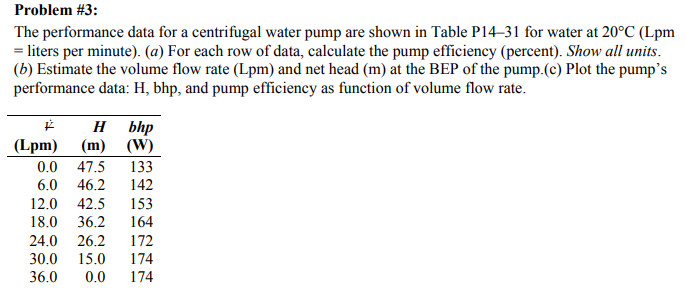Problem #3: The performance data for a centrifugal water pump are shown in Table P14-31 for water at 20°c (Lpm = liters per minute). (a) For each row of data, calculate the pump efficiency (percent). Show all units. (b) Estimate the volume flow rate (Lpm) and net head (m) at the BEP of the pump.(c) Plot the pump's performance data: H, bhp, and pump efficiency as function of volume flow rate. H bhp Lpm) (m) (W) 0.0 47.5 133 6.0...

• ### 08 % Problem 4 (25 points) A 38-in pump is installed for the system below. The...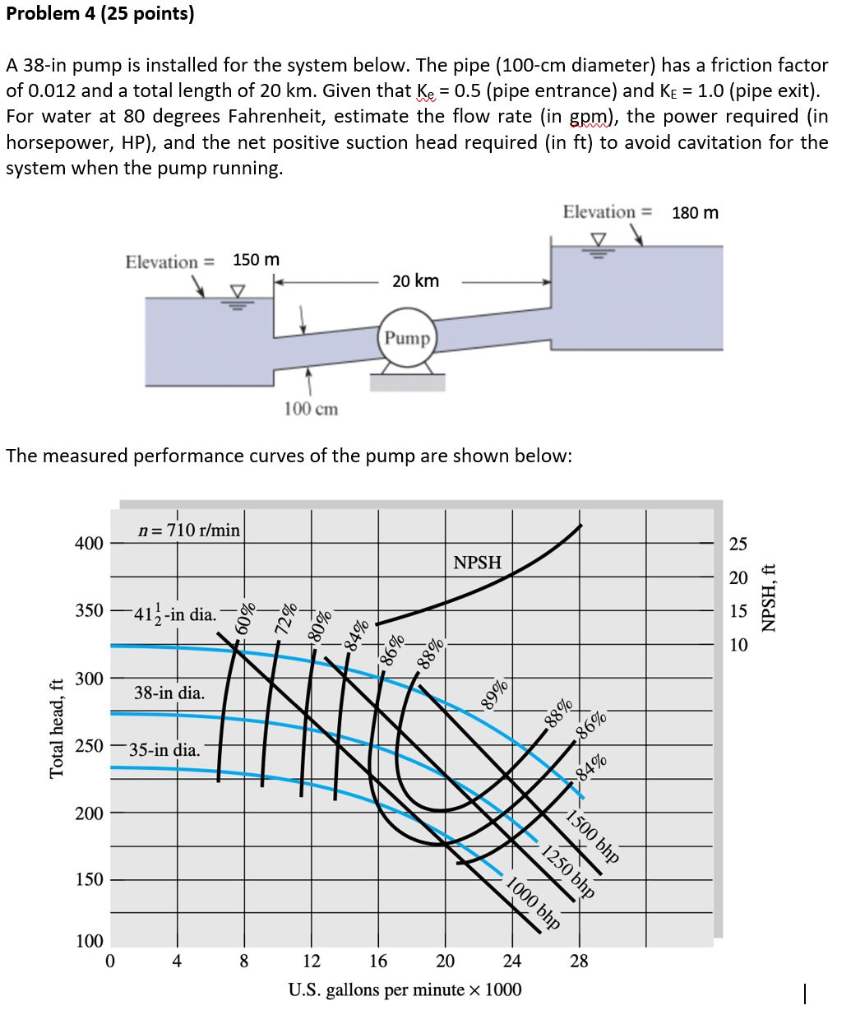08 % Problem 4 (25 points) A 38-in pump is installed for the system below. The pipe (100-cm diameter) has a friction factor of 0.012 and a total length of 20 km. Given that Ke = 0.5 (pipe entrance) and Ke = 1.0 (pipe exit). For water at 80 degrees Fahrenheit, estimate the flow rate (in gpm), the power required (in horsepower, HP), and the net positive suction head required (in ft) to avoid cavitation for the system when the...

• ### Project-Design Problem: A family of water pumps produced by a given pump manufacturer have performance and...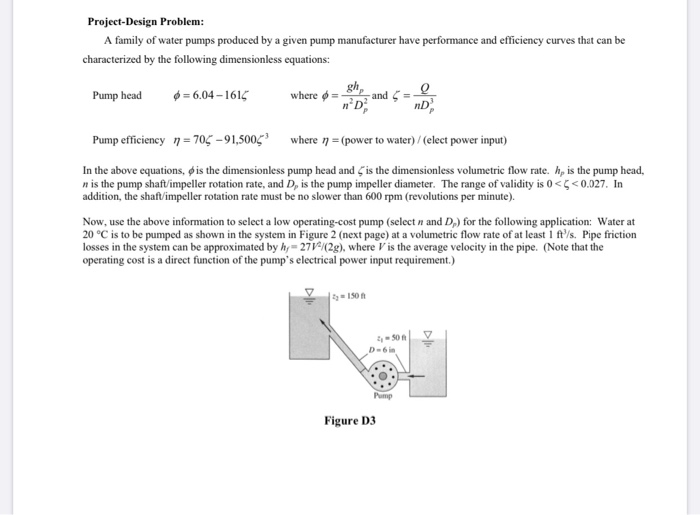Project-Design Problem: A family of water pumps produced by a given pump manufacturer have performance and efficiency curves that can be characterized by the following dimensionless equations: Pump head 6 = 6.04-1616 where and 5 nD Pump efficiency n = 705-91,5006 where n = (power to water) / (elect power input) In the above equations, is the dimensionless pump head and is the dimensionless volumetric flow rate. h, is the pump head, n is the pump shaft/impeller rotation rate, and...

• ### 3. A pump is to be used to pump water from the lower re servoir at an elevation of 10 ft The pump has a characteristic curve ft up to a reservoir shown in the graph below. What will be the volum...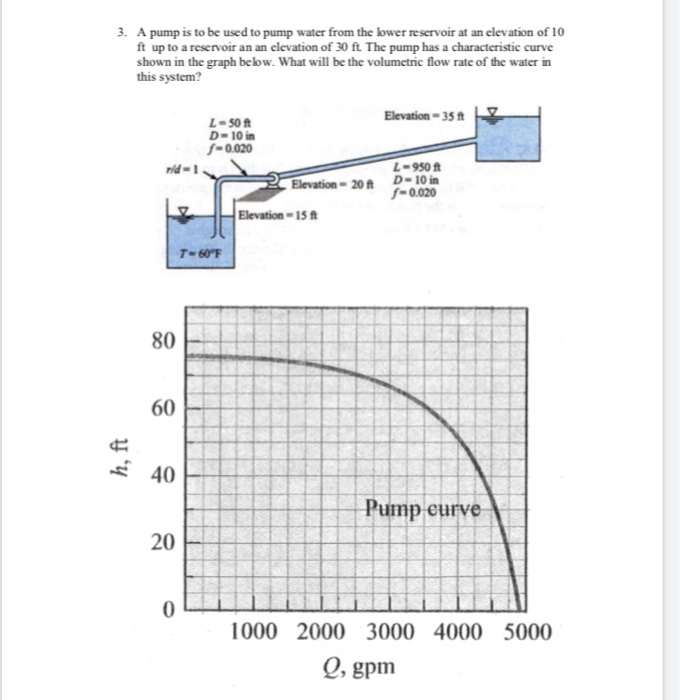3. A pump is to be used to pump water from the lower re servoir at an elevation of 10 ft The pump has a characteristic curve ft up to a reservoir shown in the graph below. What will be the volumetric flow rate of the water in this system? Elevation- 35 ft L- 50 t D-10 in -0.020 L-950 ft rid- Elevation 20A D-10 i -0.020 Elevation-15ft 80 60 40 Pump curve 20 1000 2000 3000 4000 5000 O,...

• ### A 60 horsepower pump is used to move water from a large reservoir to another at a higher elevation. Measured data show...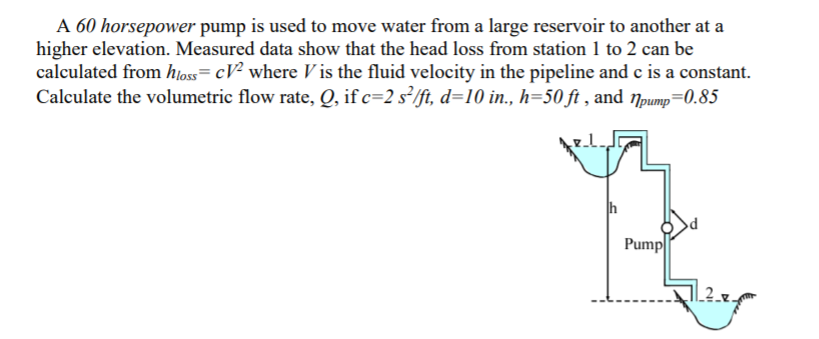A 60 horsepower pump is used to move water from a large reservoir to another at a higher elevation. Measured data show that the head loss from station 1 to 2 can be calculated from hloss= cV2 where V is the fluid velocity in the pipeline and c is a constant Calculate the volumetric flow rate, Q, if c=2 s2/ft, d=10 in., h=50 ft , and npump=0.85 h Pump A 60 horsepower pump is used to move water from a...

• ### 1. A centrifugal pump performance data are shown in the following table for water. Pump speed-250...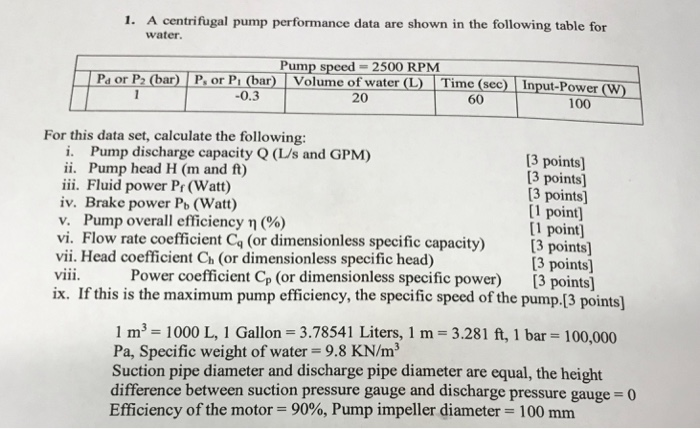Mechanical Lab II. Please answer asap with clear work. Thank you! 1. A centrifugal pump performance data are shown in the following table for water. Pump speed-2500 RPM Pa or P2 (bar) P. or Pi (bar) Volume of water(L Time (sec) Input-Power (W) -0.3 20 60 100 For this data set, calculate the following i. Pump discharge capacity Q (LUs and GPM) ii. Pump head H (m and ft) iii. Fluid power Pr (Watt) iv. Brake power Pb (Watt) v....

• ### A turbo pump transfers water from tank 1 to tank to tank 2 as shown in...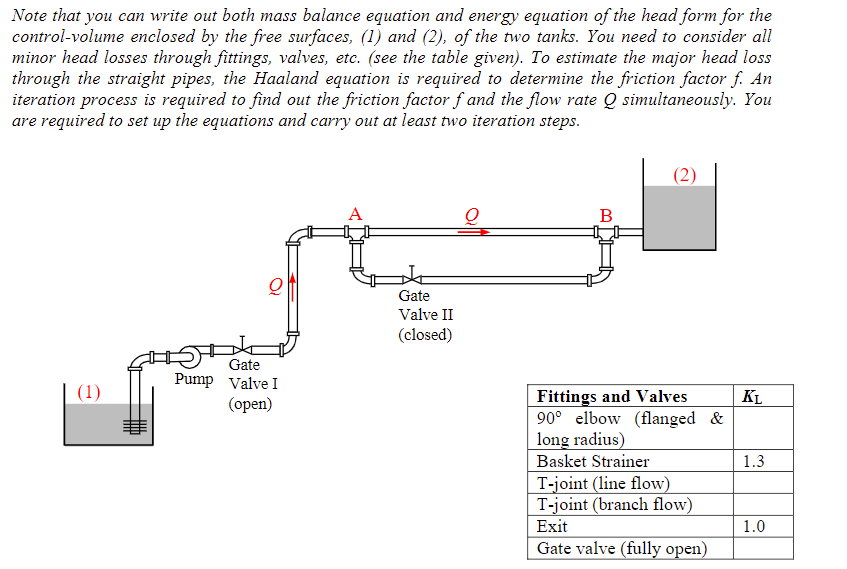A turbo pump transfers water from tank 1 to tank to tank 2 as shown in the picture below. The diameter of the piping system is D= 4in and the total length of pipes is L=620 ft. the elevation difference is Z1-Z2= 30ft. Use the chart for KL values. a) determine the flow rate (Q) b) power of the pump in HP Note that you can write out both mass balance equation and energy equation of the head form for...

• ### A turbo pump transfers water from tank 1 to tank to tank 2 as shown in...A turbo pump transfers water from tank 1 to tank to tank 2 as shown in the picture below. The diameter of the piping system is D= 4in and the total length of pipes is L=620 ft. the elevation difference is Z1-Z2= 30ft. Use the chart for KL values. a) determine the flow rate (Q) b) power of the pump in HP Note that you can write out both mass balance equation and energy equation of the head form for...

• ### A pump transports water from Tank 1 to Tank 2 through a constant-diameter piping system as...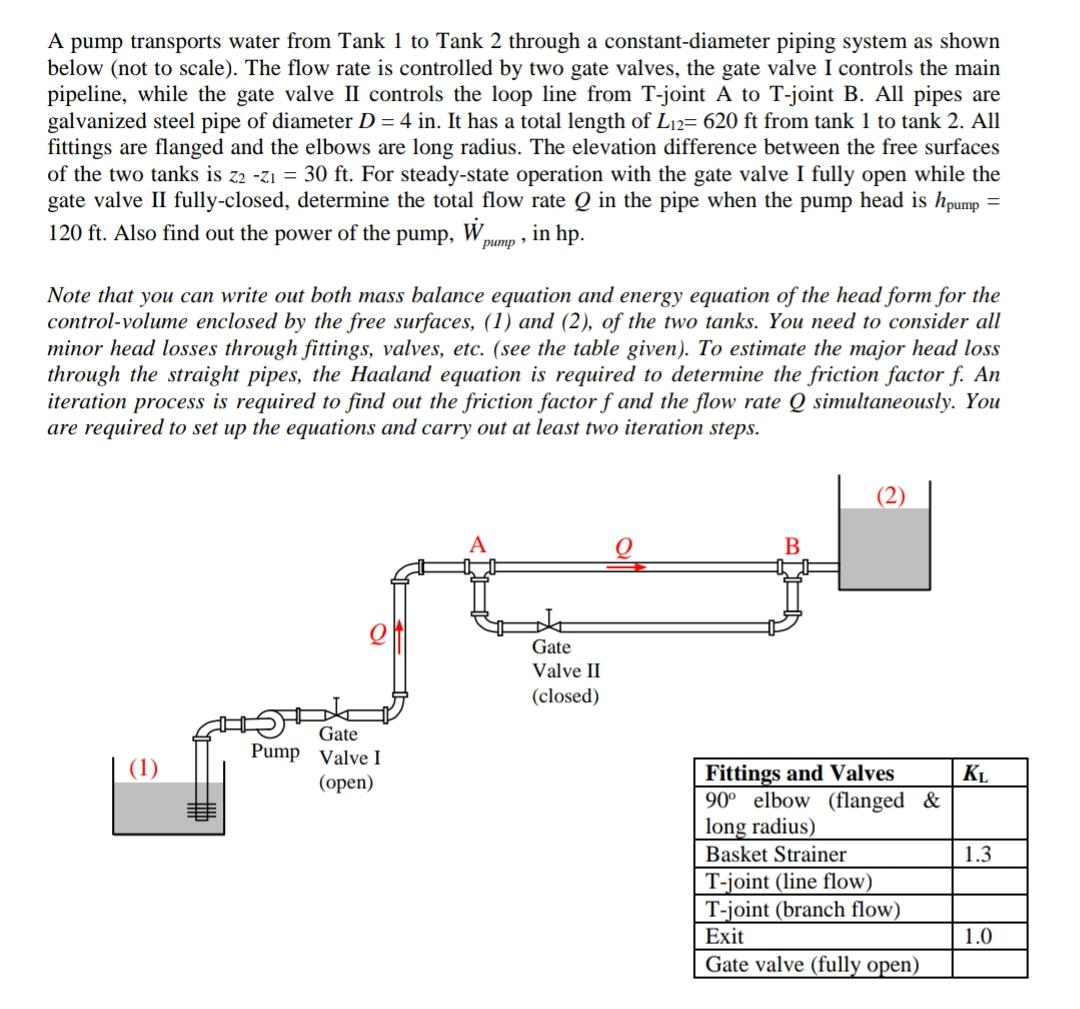A pump transports water from Tank 1 to Tank 2 through a constant-diameter piping system as shown below (not to scale). The flow rate is controlled by two gate valves, the gate valve I controls the main pipeline, while the gate valve II controls the loop line from T-joint A to T-joint B. All pipes are galvanized steel pipe of diameter D = 4 in. It has a total length of Li2= 620 ft from tank 1 to tank 2....